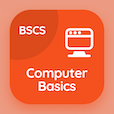Engineering Online Courses

Engineering Physics Quizzes

Engineering Physics Quiz PDF - Complete

# Sun,Earth and Moon Multiple Choice Questions p. 191

Study Sun,Earth and Moon multiple choice questions and answers, sun,earth and moon quiz answers PDF 191 to study Engineering Physics course online. Astronomical Data MCQ trivia questions, Sun,Earth and Moon Multiple Choice Questions (MCQ) for online college degrees. "Sun,Earth and Moon MCQ" PDF eBook: gauss law, zeroth law of thermodynamics, resistivity of typical insulators, inclination of planet axis to orbit, sun,earth and moon test prep for online engineering associate's degree.

"Mean density of Earth is" MCQ PDF: 1410 kg/m3, 2320 kg/m3, 5520 kg/m3, and 3340 kg/m3 to learn free online courses. Learn astronomical data questions and answers to improve problem solving skills for job assessment test.

## Sun,Earth and Moon Questions and Answers MCQs

MCQ: Mean density of Earth is

2320 kg/m3
1410 kg/m3
5520 kg/m3
3340 kg/m3

MCQ: A term used for the angle between two orbital planes is

orbital inclination
orbital reflection
linear inclination
unit inclination

MCQ: Fused Quartz has resistivity of

1012 Ωm
1016 Ωm
1016 Ωm
10 Ωm

MCQ: Thermometer which contains a bulb full of gas that is attached with one mercury manometer which contains a column, some part of which contains mercury connected to a reservoir is called

Aluminium thermometer
Gas thermometer
Mercury Thermometer
Bulb thermometer

MCQ: Law which states that electric field at point on a closed Gaussian surface to the net charge enclosed by that surface is

Newton's Second Law for a System of Particles
Gauss Law
Lenz Law

### More Quizzes from Engineering Physics Course